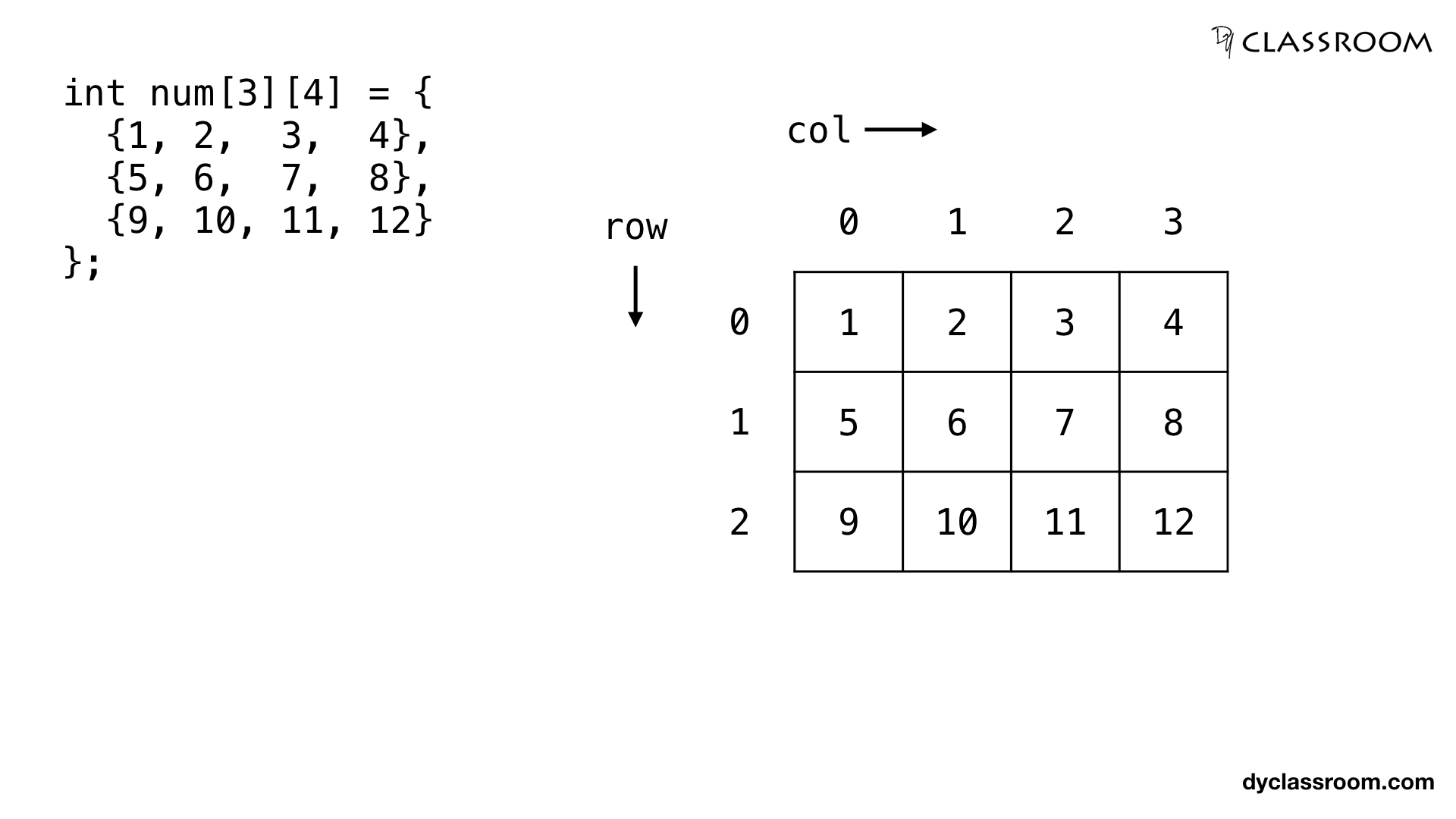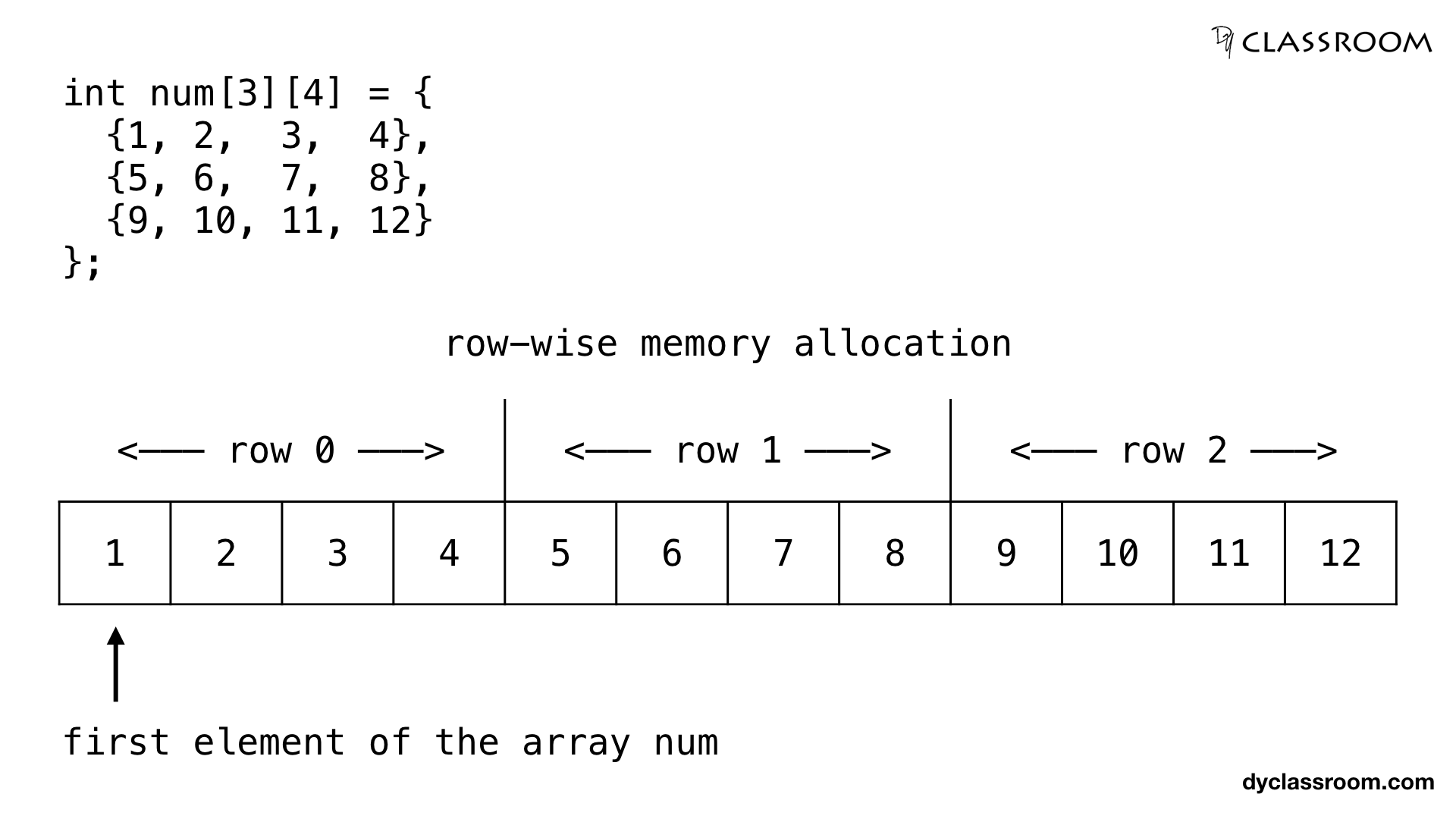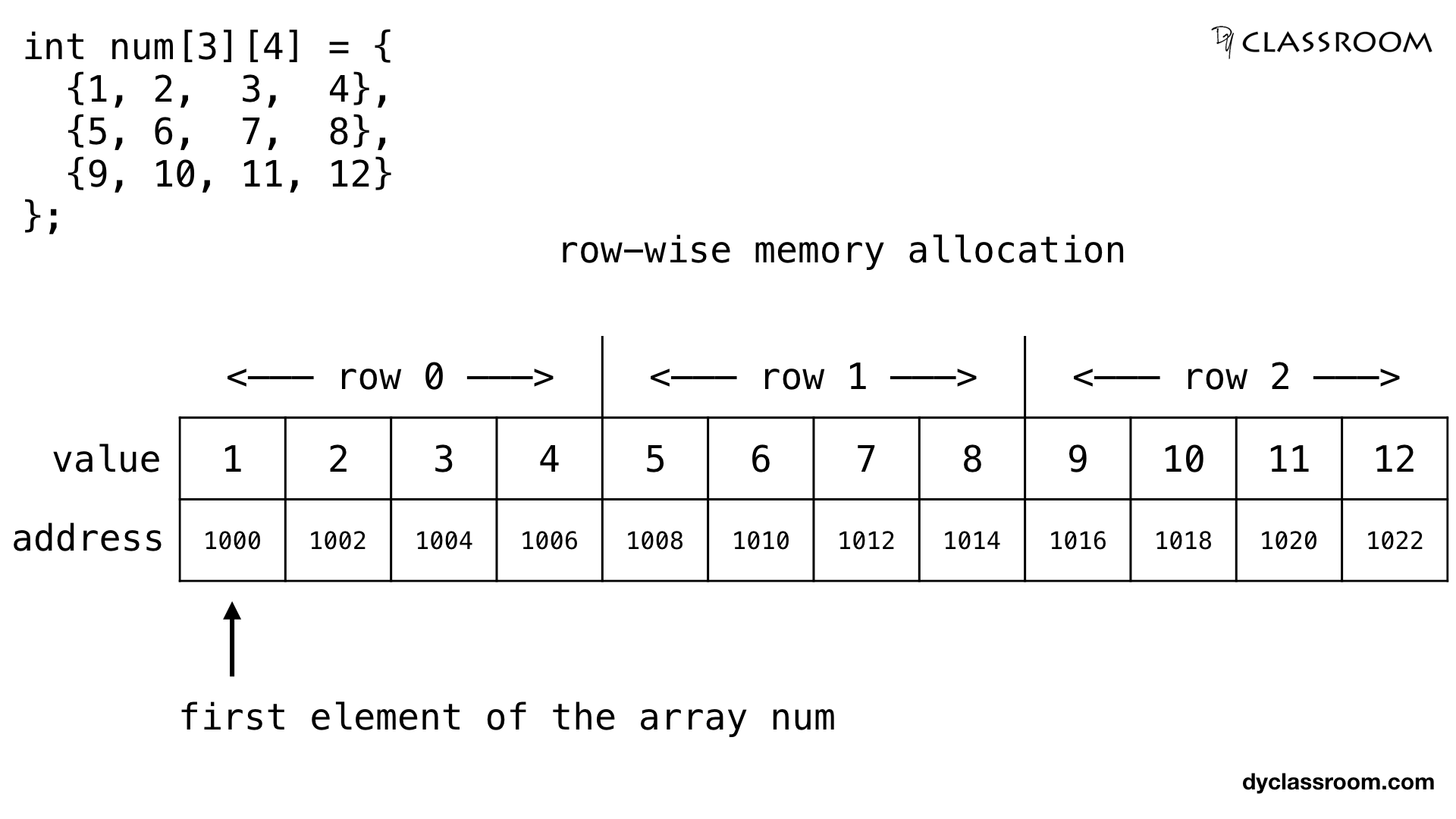# C - Pointers and Two Dimensional Array

C ProgrammingIn this tutorial we will learn to work with two dimensional arrays using pointers in C programming language.

In the previous tutorial Pointers and One Dimensional Array we learned to work with one dimensional character array. Feel free to checkout that tutorial.

## Creating a two dimensional array

To keep things simple we will create a two dimensional integer array `num` having 3 rows and 4 columns.

``````int num = {
{1, 2,  3,  4},
{5, 6,  7,  8},
{9, 10, 11, 12}
};
``````In the above image we are showing the two dimensional array having 3 rows and 4 columns.

The compiler will allocate the memory for the above two dimensional array row-wise meaning the first element of the second row will be placed after the last element of the first row.And if we assume that the first element of the array is at address 1000 and the size of type `int` is 2 bytes then the elements of the array will get the following allocated memory locations.## Create pointer for the two dimensional array

We have created the two dimensional integer array `num` so, our pointer will also be of type `int`.

We will assign the address of the first element of the array `num` to the pointer `ptr` using the address of `&` operator.

``````int *ptr = &num;
``````

## Accessing the elements of the two dimensional array via pointer

The two dimensional array `num` will be saved as a continuous block in the memory. So, if we increment the value of ptr by 1 we will move to the next block in the allocated memory.

In the following code we are printing the content of the `num` array using `for` loop and by incrementing the value of `ptr`.

``````#include <stdio.h>

int main(void) {

// 2d array
int num = {
{1, 2,  3,  4},
{5, 6,  7,  8},
{9, 10, 11, 12}
};

// pointer ptr pointing at array num
int *ptr = &num;

// other variables
int
ROWS = 3,
COLS = 4,
TOTAL_CELLS = ROWS * COLS,
i;

// print the elements of the array num via pointer ptr
for (i = 0; i < TOTAL_CELLS; i++) {
printf("%d ", *(ptr + i));
}

return 0;
}
``````

Output:

``````1 2 3 4 5 6 7 8 9 10 11 12
``````

We can compute the address of an element of the array by using the rows and columns of the array. For this we use the following formula.

``````arr[i][j] = baseAddress + [(i x no_of_cols + j) x size_of_data_type]
``````

Where, `arr` is a two dimensional array. `i` and `j` denotes the ith row and jth column of the array.

`baseAddress` denotes the address of the first element of the array. And `no_of_cols` is the total number of columns in the row.

And `size_of_data_type` is the size of the type of the pointer. If the type is `int` then size_of_data_type = 2 bytes and if the type is `char` then size_of_data_type = 1 bytes.

For example, in the case of `num` array the baseAddress = 1000, no_of_cols = 4 and size_of_data_type = 2.

So, we can compute the memory address location of the element num as follows.

``````// address of element at cell 2,3
num = baseAddress + [(i x no_of_cols + j) x size_of_data_type]
= 1000 + [(2 x 4 + 3) x 2]
= 1000 + [(8 + 3) x 2]
= 1000 + 22
= 1022
``````## Accessing the value of the two dimensional array via pointer using row and column of the array

If we want to get the value at any given row, column of the array then we can use the value at the address of `*` operator and the following formula.

``````arr[i][j] = *(ptr + (i x no_of_cols + j))
``````

Where, `arr` is a two dimensional array and `i` and `j` denotes the ith row and jth column of the array.

`ptr` holds the address of the first element of the array.

And `no_of_cols` denotes the total number of column in the row of the array.

In the following example we are finding the value at the location 2nd row and 3rd column of the array `num`.

``````// value at num where, i = 2 and j = 3
num = *(ptr + (i x no_of_cols + j))
= *(ptr + (2 x 4 + 3))
= *(ptr + 11)
``````

### Complete code

``````#include <stdio.h>

int main(void) {

// 2d array
int num = {
{1, 2,  3,  4},
{5, 6,  7,  8},
{9, 10, 11, 12}
};

int
ROWS = 3,
COLS = 4,
i, j;

// pointer
int *ptr = &num;

// print the element of the array via pointer ptr
for (i = 0; i < ROWS; i++) {
for (j = 0; j < COLS; j++) {
printf("%d ", *(ptr + i * COLS + j));
}
printf("\n");
}

return 0;
}
``````

Output:

``````1 2 3 4
5 6 7 8
9 10 11 12
``````